# NCERT Solutions for Class 6 Maths Fraction Chapter 7 Exercise 7.3 and 7.4

In this page we have NCERT Solutions for Class 6 Maths Fraction Chapter 7 Exercise 7.3 and 7.4. Hope you like them and do not forget to like , social share and comment at the end of the page.

# Exercise 7.3

Question 1
Write the fractions. Are all these fractions equivalent?
(a)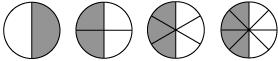(b)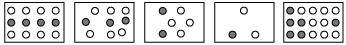a)   We can find fraction in each figure by the formula
Dark part/ total parts
½, 2/4= 1/2, 3/6= 1/2, 4/8= ½
These all are equivalent
b)
We can find fraction in each figure by the formula
Dark part/ total part
4/12=1/3, 3/9= 1/3, 2/6= 1/3, 1/3, 6/15
These all are not equivalent
Question 2
Write the fractions and pair up the equivalent fractions from each row.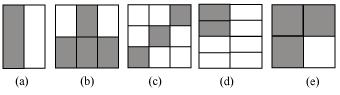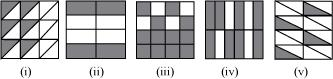We can find fraction in each figure by the formula
Dark part/ total parts
So let’s calculate the fraction
 a)  1/2 I) 6/18 =1/3 b) 4/6=2/3 ii)4/8=1/2 c) 3/9=1/3 iii)  12/16= ¾ d) 2/8=1/4 iv) 8/12= 2/3 e) 3/4 v) 4/16= 1/4
Equivalent fraction would be then
 a)  1/2 ii)4/8=1/2 b) 4/6=2/3 iv) 8/12= 2/3 c) 3/9=1/3 i) 6/18 =1/3 d) 2/8=1/4 v) 4/16= 1/4 e) 3/4 iii)  12/16= 3/4
Question 3
Replace in each of the following by the correct number:
(a)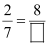(b)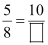(c)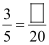(d)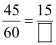(e)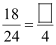To find an equivalent fraction, we may multiply/divide   both the numerator and the denominator by the same number.
a) Here in this we have to multiply by 4, so the answer would be 8/28
b) Here in this we have to multiply by 2, so the answer would be 10/16       c) Here in this we have to multiply by 4, so the answer would be 12/20
d) Here in this we have to divide by 3, so the answer would be 15/20
e) Here in this we have to divide by 6, so the answer would be 3/4
Question 4
Find the equivalent fraction of 3/5 having
(a) denominator 20
(b) numerator 9
(c) denominator 30
(d) numerator 27
To find an equivalent fraction, we may multiply/divide   both the numerator and the denominator by the same number
 a) To make denominator 20, we need to multiple both by 4 12/20 b) To make numerator 9, we need to multiple both by 3 9/15 c) To make denominator 30, we need to multiple both by 6 18/30 d) To make numerator 27, we need to multiple both by 9 27/45
Question 5
Find the equivalent fraction of 36/48 with
(a) numerator 9
(b) denominator 4
 a) To make numerator 9, we need to divide   both by 4 9/12 b) To make denominator 4, we need to divide both by 12 3/4
Question 6
Check whether the given fractions are equivalent:
(a)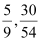(b)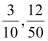(c)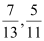a)  for the first fraction 5/9, if multiply both (numerator and denominator) by 6, then we get 30/54
So 5/9 and 30/54 are equivalent fraction
b) for the first fraction 3/10, if multiply both (numerator and denominator) by 4, then we get 12/40 and if multiply both (numerator and denominator) by 5 then we get 15/50
So 5/9 and 12/50 are not equivalent fraction
c)  All the number in both the fraction are prime numbers, so there is no chance of them being equivalent
Question 7
Reduce the following fractions to simplest form:
(a)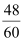(b)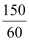(c)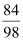(d)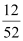(e)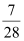To reduce the fraction to simplest form, we need to find the HCF of numerator and denominator
Let’ s takes an example of first one
 2 48,60 2 24,30 3 12,15 4,5
So HCF will be 2×2×3=12. We can find likewise all the HCF here
 a) HCF of 48 and 60 is 12, So dividing by 12 on both numerator and denominator 4/5 b) HCF of 150 and 60 is 12, So dividing by 30 on both numerator and denominator 5/2 c) HCF of 84 and 98 is 14, So dividing by 14 on both numerator and denominator 6/7 d) HCF of 12 and 52 is 4, So dividing by 4 on both numerator and denominator 3/13 e) HCF of 7 and 28 is 7, So dividing by 7 on both numerator and denominator 1/4
Question 8
Ramesh had 20 pencils, Sheela had 50 pencils and Jamaal had 80 pencils. After 4 months, Ramesh used up 10 pencils, Sheela used up 25 pencils and Jamaal used up 40 pencils. What fraction did each use up? Check if each has used up an equal fraction of her/his pencils?
Fraction of pencils used by Ramesh = Used pencil/Total pencil =10/20 =1/2

Fraction of pencils used by Sheela = Used pencil/Total pencil =25/50 =1/2

Fractions of pencils used by Jamaal = Used pencil/Total pencil =40/80 =1/2
Yes, they have all used up an equal fraction of his/her pencils.
Question 9
Match the equivalent fractions and write two more for each.
 i)   250/400 a) 2/3 ii) 180/200 b) 2/5 iii) 660/990 c) 1/2 iv) 180/360 d) 5/8 v)  220/550 e) 9/10

 i)   250/400 = 5/8(Dividing both numerator and denominator by 50) d) 5/8 ii) 180/200 =9/10(Dividing both numerator and denominator by 20) e) 9/10 iii) 660/990= 2/3(Dividing both numerator and denominator by 330) a) 2/3 iv) 180/360= 1/2(Dividing both numerator and denominator by 180) c) 1/2 v)  220/550(Dividing both numerator and denominator by 110) b) 2/5

# Exercise 7.4

Question   1
Write shaded portion as fraction. Arrange them in ascending and descending order using correct sign ‘<’, ‘=’, ‘>’ between the fractions:
(a)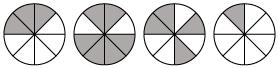(b)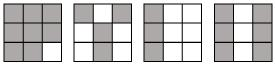(c) Show 2/6, 4/6, 8/6, 6/6 on the number line. Put appropriate signs between the fractions given.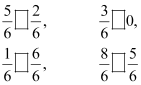The fractions represented by the shaded portions are as follows:
a) 3/8, 6/8, 4/8 1/8
b) 3/8, 4/9, 3/9, 6/9

When we compare two fraction
1) Like Fraction: if the denominator is same, the fraction with the greater numerator is greater.
2) Unlike Fraction: If the denominator is not same, we first get their equivalent fractions with a denominator which is the L.C.M of the denominators of both the fractions
The above fractions in a) and b) are like fractions as they have the same denominator.
When comparing fractions with same denominator,
So, arranging the fractions in ascending order, we have
a)  1/8   < 3/8   < 4/8 < 6/8
b)  3/9 < 4/9 < 6/9   < 8/9
c)Let us represent the fractions 2/6, 4/6, 8/6, 6/6   on the number line.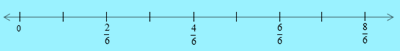Again these comparisons are between the like fractions, so numerator will dictate the sign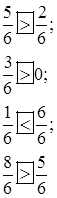Question 2
Compare the fractions and put an appropriate sign.
(a)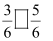(b)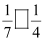(c)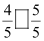(d)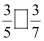a) Here the fraction is like fraction, so numerator will dictate the sign, since 3 is less than 5 < b) Here the fraction is unlike fraction, we first get their equivalent fractions with a denominator which is the L.C.M of the denominators of both the fractions LCM of 7 and 4 is 28. Equivalent Fraction for 1/7 will be 4/28 Equivalent Fraction for 1/4 will be 7/28 Now as 4 < 7 < c) Here the fraction is like fraction, so numerator will dictate the sign, since 4 is less than 5 < d) Here the fraction is unlike fraction, we first get their equivalent fractions with a denominator which is the L.C.M of the denominators of both the fractions LCM of 5 and 7 is 35. Equivalent Fraction for 3/5 will be 21/35 Equivalent Fraction for 3/7 will be 15/35 Now as 21 > 15 >
Question 4
Look at the figures and write ‘<’ or ‘>’, ‘=’ between the given pairs of fractions.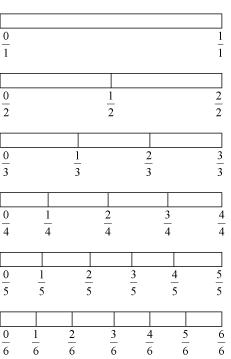(a)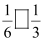(b)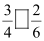(c)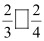(d)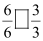(e)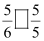a) <
b) >
c) >
d) =
e) <
Question 5
How quickly can you do this? Fill appropriate sign (‘<’, ‘=’, ‘>’)
(a)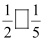(b)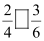(c)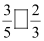(d)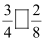(e)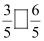(f)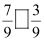(g)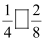(h)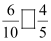(I)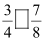(j)(k)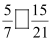a) If the numerator is same, then the smaller of the denominator fraction is bigger > b) 2/4=1/2 and 3/6=1/2 = c) These are unlike fraction, So 3/5= 9/15 2/3= 10/15 < d) ¾=3/4 2/8= 1/4 > e) Like fraction < f) Like fraction > g) ¼=1/4 2/8=1/4 = h) 6/10=3/5 4/5=4/5 < I) Unlike fraction < j) 6/10=3/5 4/5=4/5 < k) 5/7=15/21 =
Question 6
The following fractions represent just three different numbers. Separate them into three groups of equivalent fractions, by changing each one to its simplest form.
(a) 2/12
(b) 3/15
(c) 8/50
(d)16/100
(e)10/60
(f)15/75
(g)12/60
(h)16/96
(I)12/75
(j)12/75
(k)3/18
(l)4/25
Simplifying the fraction into simplest form
 i) 2/12= 1/6 ii) 3/15=1/5 iii) 8/50= 4/25 iv) 16/100= 4/25 v) 10/60=1/6 vi) 15/75=1/5 vii) 12/60=1/5 viii) 16/96=1/6 ix) 12/75=4/25 x) 12/72=1/6 xi) 3/18=1/6 xii) 4/25
 1/6 i) v) viii) x) xi) 1/5 ii) vi) vii) 4/25 iii) iv) ix) xii)
Question 7
Find answers to the following. Write and indicate how you solved them.
(a) Is 5/9 equal to 4/5?
(b) Is 9/16 equal to 5/9?
(c) Is 4/5 equal to 16/20?
(d) Is 1/15 equal to 4/30?
We need to first simplify each fraction. After simplication if they are equal, else it is not equal
a) Not equal
b) Not equal
c) equal
d) Not equal
Question 8
Ila read 25 pages of a book containing 100 pages. Lalita read 2/5 of the same book. Who read less?
Total number of pages in the book = 100 pages
Number of pages read by Ila = 25 pages
Fraction of pages read by Ila = 25/100= 1/4
Lalita read 2/5 of the same book
So basically we need to compare the fraction ¼ and 2/5
Since they are unlike fraction, we need to find LCM of 4 and 5 which is 20
So ¼ = 5/20
2/5= 8/20
Now 8 > 5
So Ila read lesser than Lalita
Question 9
Rafiq exercised for 3/6 of an hour, while Rohit exercised for ¾ of an hour. Who exercised for a longer time?
Rafiq =3/6
Rohit = ¾
We need to compare the fraction. Since these are unlike fraction, we need to calculate the LCM and 4 and 6. LCM of 4 and 6 is 12
Raft=3/6= 6/12
Rohit= ¾= 9/12
9/12 > 6/12
So clearly Rohit exercised more
Question 10
In a class A of 25 students, 20 passed in first class; in another class B of 30 students, 24 passed in first class. In which class was a greater fraction of students getting first class?
Total number of students in class A = 25
Number of students passed in first class = 20.
Fraction of students of class A who passed in first class = 20/25=4/5
Total number of students in class B = 30
Number of students passed in first class = 24.
Fraction of students of class B who passed in first class =24/30 =4/5
Both class A and B have the same fraction of students who passed in first class.

### Practice Question

Question 1 What is $\frac {1}{2} + \frac {3}{4}$ ?
A)$\frac {5}{4}$
B)$\frac {1}{4}$
C)$1$
D)$\frac {4}{5}$
Question 2 Pinhole camera produces an ?
A)An erect and small image
B)an Inverted and small image
C)An inverted and enlarged image
D)None of the above